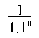xmlns:atom="http://www.w3.org/2005/Atom"

## SCHEDULE 2METHOD FOR CALCULATING MULTIPLIER

(introduced by section 3(1)(b))

1As respects each date on which, but for this Act, a payment of the casualty would have fallen due, a value shall, subject to paragraphs 2 and 3 below, be calculated, rounded to three decimal places, in accordance with the formula—where n is the period, in years, between the relevant date and the date of the payment; and the sum of all the values so calculated shall be the multiplier for the purposes of section 3(1)(b) of this Act.

2For the purposes of paragraph 1 above, for any case where the period is—

(a)less than ½ year (or is nil), the value shall be 1;

(b)½ but less than 1 year, the value shall be 0.953.

3For the purposes of reckoning n in paragraph 1 above, where the period is more than one year, n shall be the whole number of years in question (with any time in excess of that number being ignored).